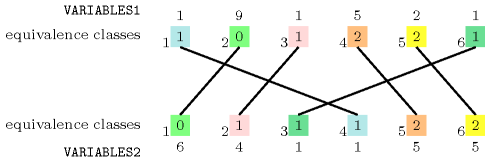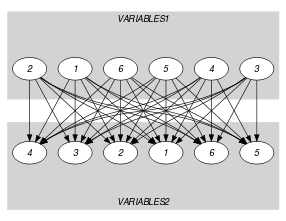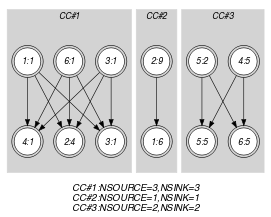## 5.339. same_modulo

Origin

Derived from $\mathrm{𝚜𝚊𝚖𝚎}$.

Constraint

$\mathrm{𝚜𝚊𝚖𝚎}_\mathrm{𝚖𝚘𝚍𝚞𝚕𝚘}\left(\mathrm{𝚅𝙰𝚁𝙸𝙰𝙱𝙻𝙴𝚂}\mathtt{1},\mathrm{𝚅𝙰𝚁𝙸𝙰𝙱𝙻𝙴𝚂}\mathtt{2},𝙼\right)$

Arguments
 $\mathrm{𝚅𝙰𝚁𝙸𝙰𝙱𝙻𝙴𝚂}\mathtt{1}$ $\mathrm{𝚌𝚘𝚕𝚕𝚎𝚌𝚝𝚒𝚘𝚗}\left(\mathrm{𝚟𝚊𝚛}-\mathrm{𝚍𝚟𝚊𝚛}\right)$ $\mathrm{𝚅𝙰𝚁𝙸𝙰𝙱𝙻𝙴𝚂}\mathtt{2}$ $\mathrm{𝚌𝚘𝚕𝚕𝚎𝚌𝚝𝚒𝚘𝚗}\left(\mathrm{𝚟𝚊𝚛}-\mathrm{𝚍𝚟𝚊𝚛}\right)$ $𝙼$ $\mathrm{𝚒𝚗𝚝}$
Restrictions
 $|\mathrm{𝚅𝙰𝚁𝙸𝙰𝙱𝙻𝙴𝚂}\mathtt{1}|=|\mathrm{𝚅𝙰𝚁𝙸𝙰𝙱𝙻𝙴𝚂}\mathtt{2}|$ $\mathrm{𝚛𝚎𝚚𝚞𝚒𝚛𝚎𝚍}$$\left(\mathrm{𝚅𝙰𝚁𝙸𝙰𝙱𝙻𝙴𝚂}\mathtt{1},\mathrm{𝚟𝚊𝚛}\right)$ $\mathrm{𝚛𝚎𝚚𝚞𝚒𝚛𝚎𝚍}$$\left(\mathrm{𝚅𝙰𝚁𝙸𝙰𝙱𝙻𝙴𝚂}\mathtt{2},\mathrm{𝚟𝚊𝚛}\right)$ $𝙼>0$
Purpose

For each integer $R$ in $\left[0,𝙼-1\right]$, let $𝑁{\mathit{1}}_{R}$ (respectively $𝑁{\mathit{2}}_{R}$) denote the number of variables of $\mathrm{𝚅𝙰𝚁𝙸𝙰𝙱𝙻𝙴𝚂}\mathtt{1}$ (respectively $\mathrm{𝚅𝙰𝚁𝙸𝙰𝙱𝙻𝙴𝚂}\mathtt{2}$) that have $R$ as a rest when divided by $𝙼$. For all $R$ in $\left[0,𝙼-1\right]$ we have that $𝑁{\mathit{1}}_{R}=𝑁{\mathit{2}}_{R}$.

Example
$\left(〈1,9,1,5,2,1〉,〈6,4,1,1,5,5〉,3\right)$

The values of the first collection $〈1,9,1,5,2,1〉$ are respectively associated with the equivalence classes $1\mathrm{mod}3=1$, $9\mathrm{mod}3=0$, $1\mathrm{mod}3=1$, $5\mathrm{mod}3=2$, $2\mathrm{mod}3=2$, $1\mathrm{mod}3=1$. Therefore the equivalence classes 0, 1, and 2 are respectively used 1, 3, and 2 times. Similarly, the values of the second collection $〈6,4,1,1,5,5〉$ are respectively associated with the equivalence classes $6\mathrm{mod}3=0$, $4\mathrm{mod}3=1$, $1\mathrm{mod}3=1$, $1\mathrm{mod}3=1$, $5\mathrm{mod}3=2$, $5\mathrm{mod}3=2$. Therefore the equivalence classes 0, 1, and 2 are respectively used 1, 3, and 2 times. Consequently the $\mathrm{𝚜𝚊𝚖𝚎}_\mathrm{𝚖𝚘𝚍𝚞𝚕𝚘}$ constraint holds. Figure 5.339.1 illustrates this correspondence.

##### Figure 5.339.1. Illustration of the correspondence between the items of the $\mathrm{𝚅𝙰𝚁𝙸𝙰𝙱𝙻𝙴𝚂}\mathtt{1}$ and of the $\mathrm{𝚅𝙰𝚁𝙸𝙰𝙱𝙻𝙴𝚂}\mathtt{2}$ collections of the Example slotTypical
 $|\mathrm{𝚅𝙰𝚁𝙸𝙰𝙱𝙻𝙴𝚂}\mathtt{1}|>1$ $\mathrm{𝚛𝚊𝚗𝚐𝚎}$$\left(\mathrm{𝚅𝙰𝚁𝙸𝙰𝙱𝙻𝙴𝚂}\mathtt{1}.\mathrm{𝚟𝚊𝚛}\right)>1$ $\mathrm{𝚛𝚊𝚗𝚐𝚎}$$\left(\mathrm{𝚅𝙰𝚁𝙸𝙰𝙱𝙻𝙴𝚂}\mathtt{2}.\mathrm{𝚟𝚊𝚛}\right)>1$ $𝙼>1$ $𝙼<$$\mathrm{𝚖𝚊𝚡𝚟𝚊𝚕}$$\left(\mathrm{𝚅𝙰𝚁𝙸𝙰𝙱𝙻𝙴𝚂}\mathtt{1}.\mathrm{𝚟𝚊𝚛}\right)$ $𝙼<$$\mathrm{𝚖𝚊𝚡𝚟𝚊𝚕}$$\left(\mathrm{𝚅𝙰𝚁𝙸𝙰𝙱𝙻𝙴𝚂}\mathtt{2}.\mathrm{𝚟𝚊𝚛}\right)$
Symmetries
• Arguments are permutable w.r.t. permutation $\left(\mathrm{𝚅𝙰𝚁𝙸𝙰𝙱𝙻𝙴𝚂}\mathtt{1},\mathrm{𝚅𝙰𝚁𝙸𝙰𝙱𝙻𝙴𝚂}\mathtt{2}\right)$ $\left(𝙼\right)$.

• Items of $\mathrm{𝚅𝙰𝚁𝙸𝙰𝙱𝙻𝙴𝚂}\mathtt{1}$ are permutable.

• Items of $\mathrm{𝚅𝙰𝚁𝙸𝙰𝙱𝙻𝙴𝚂}\mathtt{2}$ are permutable.

• An occurrence of a value $u$ of $\mathrm{𝚅𝙰𝚁𝙸𝙰𝙱𝙻𝙴𝚂}.\mathrm{𝚟𝚊𝚛}$ can be replaced by any other value $v$ such that $v$ is congruent to $u$ modulo $𝙼$.

Arg. properties

Aggregate: $\mathrm{𝚅𝙰𝚁𝙸𝙰𝙱𝙻𝙴𝚂}\mathtt{1}\left(\mathrm{𝚞𝚗𝚒𝚘𝚗}\right)$, $\mathrm{𝚅𝙰𝚁𝙸𝙰𝙱𝙻𝙴𝚂}\mathtt{2}\left(\mathrm{𝚞𝚗𝚒𝚘𝚗}\right)$, $𝙼\left(\mathrm{𝚒𝚍}\right)$.

Used in

specialisation: $\mathrm{𝚜𝚊𝚖𝚎}$ ($\mathrm{𝚟𝚊𝚛𝚒𝚊𝚋𝚕𝚎}\mathrm{mod}\mathrm{𝚌𝚘𝚗𝚜𝚝𝚊𝚗𝚝}$ replaced by $\mathrm{𝚟𝚊𝚛𝚒𝚊𝚋𝚕𝚎}$).

Keywords
Arc input(s)

$\mathrm{𝚅𝙰𝚁𝙸𝙰𝙱𝙻𝙴𝚂}\mathtt{1}$ $\mathrm{𝚅𝙰𝚁𝙸𝙰𝙱𝙻𝙴𝚂}\mathtt{2}$

Arc generator
$\mathrm{𝑃𝑅𝑂𝐷𝑈𝐶𝑇}$$↦\mathrm{𝚌𝚘𝚕𝚕𝚎𝚌𝚝𝚒𝚘𝚗}\left(\mathrm{𝚟𝚊𝚛𝚒𝚊𝚋𝚕𝚎𝚜}\mathtt{1},\mathrm{𝚟𝚊𝚛𝚒𝚊𝚋𝚕𝚎𝚜}\mathtt{2}\right)$

Arc arity
Arc constraint(s)
$\mathrm{𝚟𝚊𝚛𝚒𝚊𝚋𝚕𝚎𝚜}\mathtt{1}.\mathrm{𝚟𝚊𝚛}\mathrm{mod}𝙼=\mathrm{𝚟𝚊𝚛𝚒𝚊𝚋𝚕𝚎𝚜}\mathtt{2}.\mathrm{𝚟𝚊𝚛}\mathrm{mod}𝙼$
Graph property(ies)
 $•\text{for}\text{all}\text{connected}\text{components:}$$\mathrm{𝐍𝐒𝐎𝐔𝐑𝐂𝐄}$$=$$\mathrm{𝐍𝐒𝐈𝐍𝐊}$ $•$$\mathrm{𝐍𝐒𝐎𝐔𝐑𝐂𝐄}$$=|\mathrm{𝚅𝙰𝚁𝙸𝙰𝙱𝙻𝙴𝚂}\mathtt{1}|$ $•$$\mathrm{𝐍𝐒𝐈𝐍𝐊}$$=|\mathrm{𝚅𝙰𝚁𝙸𝙰𝙱𝙻𝙴𝚂}\mathtt{2}|$

Graph model

Parts (A) and (B) of Figure 5.339.2 respectively show the initial and final graph associated with the Example slot. Since we use the $\mathrm{𝐍𝐒𝐎𝐔𝐑𝐂𝐄}$ and $\mathrm{𝐍𝐒𝐈𝐍𝐊}$ graph properties, the source and sink vertices of the final graph are stressed with a double circle. Since there is a constraint on each connected component of the final graph we also show the different connected components. Each of them corresponds to an equivalence class according to the arc constraint. The $\mathrm{𝚜𝚊𝚖𝚎}_\mathrm{𝚖𝚘𝚍𝚞𝚕𝚘}$ constraint holds since:

• Each connected component of the final graph has the same number of sources and of sinks.

• The number of sources of the final graph is equal to $|\mathrm{𝚅𝙰𝚁𝙸𝙰𝙱𝙻𝙴𝚂}\mathtt{1}|$.

• The number of sinks of the final graph is equal to $|\mathrm{𝚅𝙰𝚁𝙸𝙰𝙱𝙻𝙴𝚂}\mathtt{2}|$.

##### Figure 5.339.2. Initial and final graph of the $\mathrm{𝚜𝚊𝚖𝚎}_\mathrm{𝚖𝚘𝚍𝚞𝚕𝚘}$ constraint(a)(b)
Signature

Since the initial graph contains only sources and sinks, and since isolated vertices are eliminated from the final graph, we make the following observations:

• Sources of the initial graph cannot become sinks of the final graph,

• Sinks of the initial graph cannot become sources of the final graph.

From the previous observations and since we use the $\mathrm{𝑃𝑅𝑂𝐷𝑈𝐶𝑇}$ arc generator on the collections $\mathrm{𝚅𝙰𝚁𝙸𝙰𝙱𝙻𝙴𝚂}\mathtt{1}$ and $\mathrm{𝚅𝙰𝚁𝙸𝙰𝙱𝙻𝙴𝚂}\mathtt{2}$, we have that the maximum number of sources and sinks of the final graph is respectively equal to $|\mathrm{𝚅𝙰𝚁𝙸𝙰𝙱𝙻𝙴𝚂}\mathtt{1}|$ and $|\mathrm{𝚅𝙰𝚁𝙸𝙰𝙱𝙻𝙴𝚂}\mathtt{2}|$. Therefore we can rewrite $\mathrm{𝐍𝐒𝐎𝐔𝐑𝐂𝐄}=|\mathrm{𝚅𝙰𝚁𝙸𝙰𝙱𝙻𝙴𝚂}\mathtt{1}|$ to $\mathrm{𝐍𝐒𝐎𝐔𝐑𝐂𝐄}\ge |\mathrm{𝚅𝙰𝚁𝙸𝙰𝙱𝙻𝙴𝚂}\mathtt{1}|$ and simplify $\underline{\overline{\mathrm{𝐍𝐒𝐎𝐔𝐑𝐂𝐄}}}$ to $\overline{\mathrm{𝐍𝐒𝐎𝐔𝐑𝐂𝐄}}$. In a similar way, we can rewrite $\mathrm{𝐍𝐒𝐈𝐍𝐊}=|\mathrm{𝚅𝙰𝚁𝙸𝙰𝙱𝙻𝙴𝚂}\mathtt{2}|$ to $\mathrm{𝐍𝐒𝐈𝐍𝐊}\ge |\mathrm{𝚅𝙰𝚁𝙸𝙰𝙱𝙻𝙴𝚂}\mathtt{2}|$ and simplify $\underline{\overline{\mathrm{𝐍𝐒𝐈𝐍𝐊}}}$ to $\overline{\mathrm{𝐍𝐒𝐈𝐍𝐊}}$.